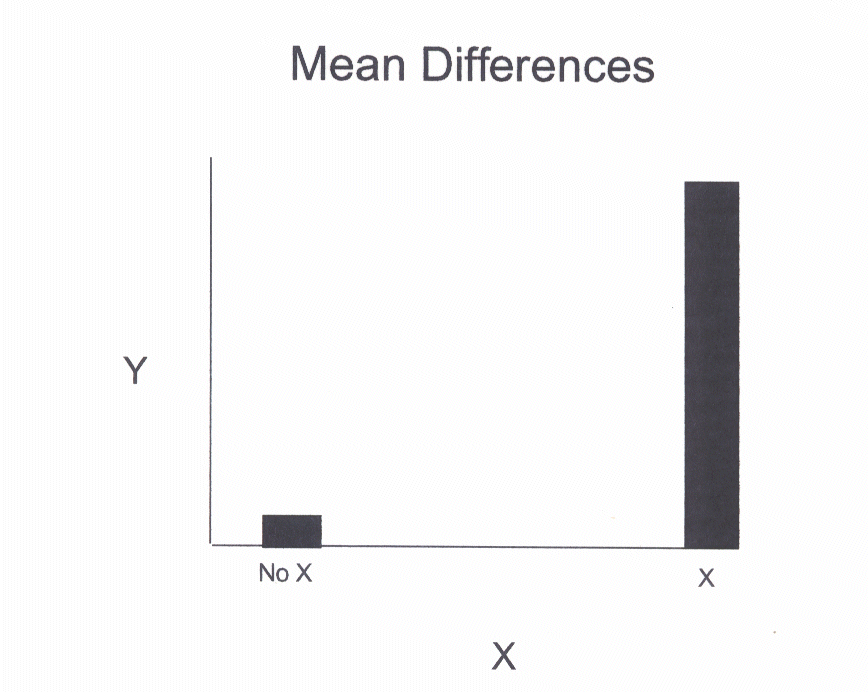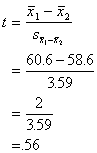Lecture 15
Point-biserial correlation, Phi, & Cramer's V

Differences and Relationships
The correlation coefficient is a measure of how two variables are related. t-tests examine how two groups are different. What if I told you these two types of questions are really the same question?

Examine the following histogram. This histogram shows a large difference in the dependent variable, y, between the groups No X and X. The mean is much higher for the X than the No X group.This is an example in which X has two groups. Now imagine that there are several values (levels or groups) for variable X: No X, a little X, some X, a lot of X, all of X. For instance, what if instead of having a study with no drug vs. drug, there is a study in which several levels of dosage used (e.g., 0mg, 5mg, 10mg, etc). Then there would be several intermittent values of X. The following graph shows one potential outcome of the study in which there are several levels of X.In this second graph, we see that as the value of X increases, the value of Y increases. That is the definition of a relationship. In other words, there is a correlation between X and Y.

The same holds true for the first figure in which there were only two values of X. As X increases, Y increases. So both graphs demonstrate that there is a relationship between X and Y. At the same time, we can see that both graphs demonstrate that the values of Y differ for the values of X. So, a difference between values of X is the same as a relationship between X and Y.

Point-biserial Correlation
A point-biserial correlation is simply the correlation between one dichotmous variable and one continuous variable. It turns out that this is a special case of the Pearson correlation. So computing the special point-biserial correlation is equivalent to computing the Pearson correlation when one variable is dichotmous and the other is continuous.

Because we know that differences are the same as correlations now, we can show that the t-test for two groups is the same as the correlation between the grouping or independent variable (X) and the dependent variable (Y). Let's go back to our last t-test example and check this out. In this example, we were comparing first and last siblings to see who was more introverted.

 First or Last Sibling Introversion 1 65 1 48 1 63 1 52 1 61 1 53 1 63 1 70 1 65 1 66 2 61 2 42 2 66 2 52 2 47 2 58 2 65 2 62 2 64 2 69

When we conducted the t-test we got the following results:This t-value was non-significant, indicating no significant differences between the groups.

If we compute a correlation between the grouping variable (sibling) and the the introversion score for this same data, the r= -.13 (I magically arrived at this figure with some assistance from my friend Hal). If we plug this into the t-test formula used for testing the significance of a correlation, we would get this:which is the very same t-value from the difference test above. The correlation test (also nonsignificant) indicates that there is no relationship between the sibling group and the introversion score.

So, a difference is a correlation.

Phi
There is another special case of correlation called "phi" (or
f, the Greek letter f ). Phi represents the correlation between two dichotmous variables. As with the point-biserial, computing the Pearson correlation for two dichotomous variables is the same as the phi.

Similar to the t-test/correlation equivalence, the relationship between two dichotomous variables is the same as the difference between two groups when the dependent variable is dichotmous. The appropriate test to compare group differences with a dichotmous outcome is the chi-square statistic. And, we can also show that the test of the phi coefficient is equivalent to the chi-square test.

Remember, one of the ways chi-square is interpreted is as a test of independence. The test of independence refers to whether or not two varables are related. If two variables are related, they are correlated.

So, when we conduct a chi-square test, and we want to have a rough estimate of how strongly related the two variables are, we can examine phi. Squaring phi will give you the approximate amount of shared variance between the two variables, as does r-square.

Cramer's V
Cramer's V is used to examine the association between two categorical variables when there is more than a 2 X 2 contingency (e.g., 2 X 3). In these more complicated designs, phi is not appropriate, but Cramer's statistic is. Cramer's V represents the association or correlation between two variables. I've also seen this statistic referred to as "Cramers Phi".